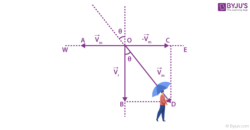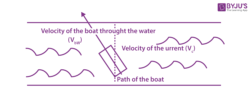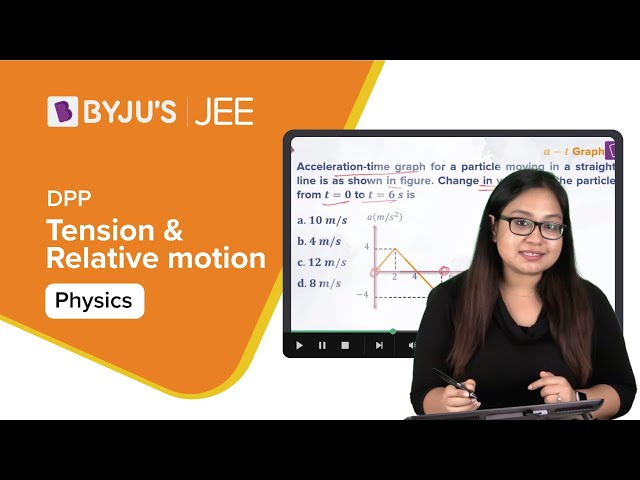Win up to 100% scholarship on Aakash BYJU'S JEE/NEET courses with ABNAT Win up to 100% scholarship on Aakash BYJU'S JEE/NEET courses with ABNAT

# Relative Motion

If you look outside the window of a moving train, you will observe that another train lying stationary appears to be moving in a backward direction. How does a stationary train seem to move? Behind it, there lies a very important concept of relative motion, which will help us understand why objects appear to move differently with different frames.

## What Is Relative Motion?

The concept of reference frames was first introduced to discuss relative motion in one or more dimensions. When we say an object has a certain velocity, then this velocity is with respect to some frame that is known as the reference frame. In everyday life, when we measure the velocity of an object, the reference frame is taken to be the ground or the earth.

For example, if you are travelling in a train and the train is moving at a speed of 100 km/hr, then your speed according to another passenger sitting on that train is zero. According to him, you are not moving. But if someone observes you from outside the train, standing on the ground, according to him, you are moving at 100 km/hr as you are on the train and the train is moving at 100 km/hr.

Here, the motion observed by the observer depends on the location (frame) of the observer. This type of motion is called relative motion.

## Relative Velocity

The relative velocity of an object A with respect to object B is the rate of change of position of the object A with respect to object B.

If VA and VB be the velocities of objects A and B with respect to the ground, then

The relative velocity of A with respect to B is VAB = VA – VB

The relative velocity of B with respect to A is VBA = VB – VA

## Relative Motion in One Dimension

In one-dimensional motion, objects move in a straight line. So, there are only two possible cases:

• Objects are moving in the same direction
• Objects are moving in the opposite direction

Again take the example of a man sitting on the train if the train is moving at 100 km/hr forward. Then according to the man sitting on the train, the trees outside are moving backwards at 100 km/hr.

This is because from the man’s point of view, the outside environment is moving in the opposite direction to the train with the same velocity.

So, for all types of questions, if you have to find the velocity of A with respect to B, then assume that B is at rest and give the velocity of B to A in the opposite direction.

## Relative Motion in One Dimension – Video Lesson## Relative Motion in Two Dimensions – Video Lesson## Relative Motion in Two Dimensions

The same concept will be applicable in two-dimensional motion. If you have to find the velocity of A with respect to B, assume that B is at rest and give the velocity of B to A in the opposite direction.

Let us consider two objects A and B which are moving with velocities Va and Vb with respect to some common frame of reference, say, with respect to the ground or the earth. We have to find the velocity of A with respect to B, so assume that B is at rest and give the velocity of B to A in the opposite direction.

Vab = va – vb

Similarly, for the velocity of object B with respect to A, assume that A is at rest and give the velocity of A to B in the opposite direction.

Vba = vb – va

## Relative Motion Problems

1) Two bodies A and B are travelling at the same speed 100 km/hr in opposite directions. Find the relative velocity of body A with respect to body B and relative velocity of body B with respect to body A.The relative velocity of A w.r.t. B is VAB = VA – VB

= 100- (-100)

= 200 km/hr

Relative velocity of B w.r.t. A is VBA = VB – VA

= -100 – (100)

= -200 km/hr (-ve means towards left)

In the same question, if both bodies are moving in the same direction with the same speed then,

The relative velocity of A with respect to B is VAB = VA – VB

= 100-100

= 0

The relative velocity of B with respect to A is VBA = VB – VA

= 100-100

= 0

That means A is at rest with respect to B and B is at rest with respect to A, but both are moving at 100 km/hr with respect to the ground.

2) Find the relative velocity of rain with respect to the moving man:Here,

$$\begin{array}{l}\text{the man is walking towards the west with velocity}\ \vec{V_{m}}\end{array}$$
$$\begin{array}{l}\text{and the rain is falling vertically downward with velocity}\ \vec{V_{r}}\end{array}$$

So, the relative velocity of rain w.r.t. man is

$$\begin{array}{l}\vec{V_{m}}=\vec{V_{r}}\end{array}$$

We know that the magnitude of the vector difference is given by,

$$\begin{array}{l}Vrm =\sqrt{V_{r}^{2}+V_{m}^{2}+2V_{r}V_{m}cos90^{0}}= \sqrt{V_{r}^{2}+V_{m}^{2}}\end{array}$$

From the diagram,

$$\begin{array}{l}\overline{Vm}\ \text{is the hypotenuse of the triangle.}\end{array}$$
$$\begin{array}{l}\text{If}\ \theta\ \text{is the angle which}\ \overline{Vrm}\ \text{makes with the vertical direction then,}\end{array}$$

tan θ = BD/OB = Vm / Vr

In the above case, if the man wants to protect himself from the rain, he should hold his umbrella in the direction of the relative velocity of rain with respect to the man, i.e., the umbrella should be held making an angle θ

$$\begin{array}{l}(\theta =tan^{-1}\frac{V_{m}}{V_{r}})\end{array}$$
west of vertical is the angle of the umbrella from the vertical.

3) Boat and river problem. Find the velocity of the boat with respect to the river.

Here, the velocity of the boat with respect to the water or the velocity of the boat in still water is given. If the observer is observing the motion from the ground, then the velocity of the boat with respect to the ground is equal to the velocity of the boat in still water plus the velocity of the water.i.e., the velocity of the boat with respect to the ground = velocity of the boat in still water + velocity of the water with respect to the ground

VBG = V BW + VWG

## Relative Motion Problem Videos

### Pseudo Force & Relative motion### Tension and Relative Motion## Frequently Asked Questions on Relative Motion

Q1

### What is the difference between absolute motion and relative motion?

The object is said to be in absolute motion when viewed from a fixed point of observation (there is no change in the position of an observer).

When there is no fixed point of view, the motion of an item is called relative motion (the position of the observer changes with respect to time).

Q2

### Why is motion always relative?

Motion is always within a certain frame of reference, therefore, motion is always relative.

Q3

### Give an example of relative motion.

Assume you’re jogging side by side with a friend. Between you and your friend, there is no relative motion. If you pass by someone who is standing stationary, you will have relative motion with respect to that individual, but not compared to your jogging friend. As a result, motion is relative.

Test Your Knowledge On Relative Motion!